# Law of the iterated logarithm

2010 Mathematics Subject Classification: Primary: 60F10 Secondary: 60F15 [MSN][ZBL]

A limit theorem in probability theory which is a refinement of the strong law of large numbers. Letbe a sequence of random variables and let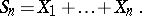For simplicity one assumes that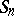has zero median for each. While the theorem on the strong law of large numbers deals with conditions under which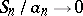almost surely (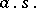) for, where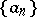is a sequence of numbers, the theorem on the law of the iterated logarithm deals with sequences of numbers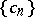such that(1)

or(2)

Relation (1) is equivalent to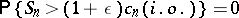andfor any, where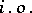denotes infinitely often.

Relations of the form of (1) and (2) hold under more restrictive conditions than the estimates implied by the strong law of large numbers. If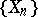is a sequence of independent random variables having identical distributions with mathematical expectations equal to zero, then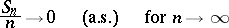(Kolmogorov's theorem); if the additional condition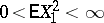is satisfied, then one has the stronger relation (2), in which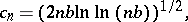where(the Hartman–Wintner theorem).

The first theorem of general type on the law of the iterated logarithm was the following result obtained by A.N. Kolmogorov [Ko]. Letbe a sequence of independent random variables with mathematical expectations equal to zero and with finite variances, and let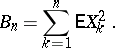If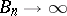forand if there exists a sequence of positive constantssuch thatthen (1) and (2) are satisfied forIn the particular case whereis a sequence of independent random variables having identical distributions with two possible values, this assertion was derived by A.Ya. Khinchin [Kh]. J. Marcinkiewicz and A. Zygmund [MZ] showed that under the conditions of Kolmogorov's theorem one cannot replaceby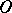. W. Feller [F] examined a generalization of Kolmogorov's law of the iterated logarithm for sequences of independent bounded non-identically distributed random variables. See [S] for other generalizations of the law; there is also the following result (see [S2]), which is related to the Hartman–Wintner theorem: Ifis a sequence of independent random variables having identical distributions with infinite variances, thenThe results obtained on the law of the iterated logarithm for sequences of independent random variables have served as a starting point for numerous researches on the applicability of this law to sequences of dependent random variables and vectors and to random processes.

How to Cite This Entry:
Law of the iterated logarithm. Encyclopedia of Mathematics. URL: http://encyclopediaofmath.org/index.php?title=Law_of_the_iterated_logarithm&oldid=26553
This article was adapted from an original article by V.V. Petrov (originator), which appeared in Encyclopedia of Mathematics - ISBN 1402006098. See original article